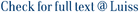The All-Pairs Min-Cut problem (aka All-Pairs Max-Flow) asks to compute a minimum s-t cut (or just its value) for all pairs of vertices s, t. We study this problem in directed graphs with unit edge/vertex capacities (corresponding to edge/vertex connectivity). Our focus is on the k-bounded case, where the algorithm has to find all pairs with min-cut value less than k, and report only those. The most basic case k = 1 is the Transitive Closure (TC) problem, which can be solved in graphs with n vertices and m edges in time O(mn) combinatorially, and in time O(nω) where ω &lt; 2.38 is the matrix-multiplication exponent. These time bounds are conjectured to be optimal. We present new algorithms and conditional lower bounds that advance the frontier for larger k, as follows: A randomized algorithm for vertex capacities that runs in time O((nk)ω). This is only a factor kω away from the TC bound, and nearly matches it for all k = no(1). Two deterministic algorithms for edge capacities (which is more general) that work in DAGs and further reports a minimum cut for each pair. The first algorithm is combinatorial (does not involve matrix multiplication) and runs in time O(2O(k2) · mn). The second algorithm can be faster on dense DAGs and runs in time O((k log n)4k+o(k) · nω). Previously, Georgiadis et al. [ICALP 2017], could match the TC bound (up to no(1) factors) only when k = 2, and now our two algorithms match it for all k = o(√log n) and k = o(log log n). The first super-cubic lower bound of nω−1−o(1)k2 time under the 4-Clique conjecture, which holds even in the simplest case of DAGs with unit vertex capacities. It improves on the previous (SETH-based) lower bounds even in the unbounded setting k = n. For combinatorial algorithms, our reduction implies an n2−o(1)k2 conditional lower bound. Thus, we identify new settings where the complexity of the problem is (conditionally) higher than that of TC. Our three sets of results are obtained via different techniques. The first one adapts the network coding method of Cheung, Lau, and Leung [SICOMP 2013] to vertex-capacitated digraphs. The second set exploits new insights on the structure of latest cuts together with suitable algebraic tools. The lower bounds arise from a novel reduction of a different structure than the SETH-based constructions.

Faster algorithms for all-pairs bounded min-cuts / Abboud, A.; Georgiadis, L.; Italiano, Giuseppe Francesco; Krauthgamer, R.; Parotsidis, N.; Trabelsi, O.; Uznanski, P.; Wolleb-Graf, D.. - Leibniz International Proceedings in Informatics, LIPIcs, (2019), pp. 1-15. (46th International Colloquium on Automata, Languages, and Programming, ICALP 2019, 2019). [10.4230/LIPIcs.ICALP.2019.7].

### Faster algorithms for all-pairs bounded min-cuts

#### Abstract

The All-Pairs Min-Cut problem (aka All-Pairs Max-Flow) asks to compute a minimum s-t cut (or just its value) for all pairs of vertices s, t. We study this problem in directed graphs with unit edge/vertex capacities (corresponding to edge/vertex connectivity). Our focus is on the k-bounded case, where the algorithm has to find all pairs with min-cut value less than k, and report only those. The most basic case k = 1 is the Transitive Closure (TC) problem, which can be solved in graphs with n vertices and m edges in time O(mn) combinatorially, and in time O(nω) where ω < 2.38 is the matrix-multiplication exponent. These time bounds are conjectured to be optimal. We present new algorithms and conditional lower bounds that advance the frontier for larger k, as follows: A randomized algorithm for vertex capacities that runs in time O((nk)ω). This is only a factor kω away from the TC bound, and nearly matches it for all k = no(1). Two deterministic algorithms for edge capacities (which is more general) that work in DAGs and further reports a minimum cut for each pair. The first algorithm is combinatorial (does not involve matrix multiplication) and runs in time O(2O(k2) · mn). The second algorithm can be faster on dense DAGs and runs in time O((k log n)4k+o(k) · nω). Previously, Georgiadis et al. [ICALP 2017], could match the TC bound (up to no(1) factors) only when k = 2, and now our two algorithms match it for all k = o(√log n) and k = o(log log n). The first super-cubic lower bound of nω−1−o(1)k2 time under the 4-Clique conjecture, which holds even in the simplest case of DAGs with unit vertex capacities. It improves on the previous (SETH-based) lower bounds even in the unbounded setting k = n. For combinatorial algorithms, our reduction implies an n2−o(1)k2 conditional lower bound. Thus, we identify new settings where the complexity of the problem is (conditionally) higher than that of TC. Our three sets of results are obtained via different techniques. The first one adapts the network coding method of Cheung, Lau, and Leung [SICOMP 2013] to vertex-capacitated digraphs. The second set exploits new insights on the structure of latest cuts together with suitable algebraic tools. The lower bounds arise from a novel reduction of a different structure than the SETH-based constructions.
##### Scheda breve Scheda completa Scheda completa (DC)2019
All-pairs min-cut; Directed graphs; Fine-grained complexity; K-reachability; Network coding
File in questo prodotto:
File
LIPIcs-ICALP-2019-7.pdf

Open Access

Tipologia: Versione dell'editore
Licenza: Creative commons
Dimensione 628.88 kB
Utilizza questo identificativo per citare o creare un link a questo documento: `https://hdl.handle.net/11385/192099`
•19
•ND